# Regularization: A Fix To Overfitting In Machine Learning

Machine Learning and Deep Learning are some of the fanciest terms in industries these days. People are getting amazed with the view of how can we even make machines, non-living objects, learn? If we ever have explored this area, we might have learned that Machine Learning algorithms try to reduce the errors between the “target variables” and the “predicted variables” for any observation. For example, suppose our machine learning algorithm is designed to say whether a Cat is present in a given image or not. For this, our ML model will read the image and say that it is confident that it contains a Cat. We as a human can see the image and identify whether the machine is right or wrong. In the above example, the target variable was “Presence of cat”. If presence was true and the machine also said it is true, then the model has learned better and vice-versa.

So, what exactly machine did for this prediction?

At the time of learning, machines made some wrong predictions, but an error function was internally associated. Whenever it made some error, a penalty got imposed. To avoid such penalties, our machines learned what they should predict. We can also say it tried to reduce the overall penalty. Hence, we can call ML algorithms an error-reducing approach. As we are trying to reduce the error function, we can also call the error function a cost function.
We must know why machine learning is also called an optimization problem as we optimize our cost function.

#### Key takeaways from this blog

After going through this blog, we will have a thorough understanding of

1. What are Underfitting, Overfitting, and Accurate fitting?
2. What is regularization?
3. How does regularization cure overfitting?
4. Mathematical logic behind regularization.
5. What are L1 and L2 regularization?

So let’s start without any further delay. Before going any further ahead, let’s quickly define three terms,

1. Underfitting
2. Overfitting
3. Appropriate fitting

Suppose we train a regression model for some problem statement, and is our performance measurement based on which we decide whether our model is learning better. The unique thing about that problem statement is, any human can achieve R² = 1. We are using Machine Learning to mimic the intelligence of that human.

Let’s give a name to the error made by humans as human error that is close to zero. The training error will be the error made by the machine learning model on the train data, and similarly, the test error will be the error made upon test data.

### Underfitting

Here, the machine learning model performs poorly on training data

#### How to detect Underfitting?

To know whether a model is underfitting or not, check the gap between human error and training error. If this gap is huge, the ML model is underfitting.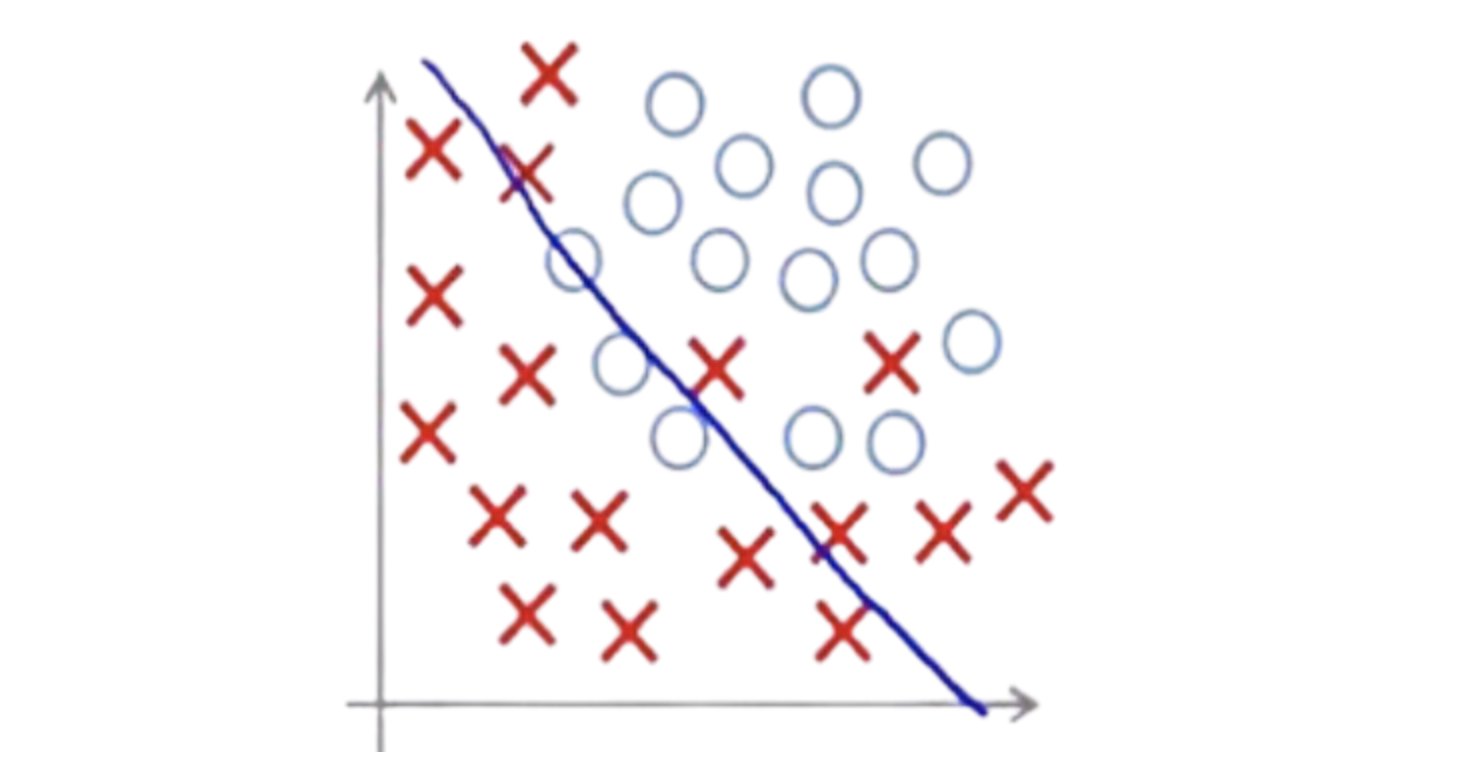#### Possible reasons and cures for underfitting

ML model is not able to learn up to the mark. The reasons could be,

• Input attributes and the target variable are highly uncorrelated.
• The pattern is complex, and the model is not capable of learning those complex patterns.

In such scenarios, generally increasing data does not help. Either we have to add, extract or engineer features to make the model learn. Or, increasing the complexity of the model can also help. In the case of neural networks, the complexity of models increases with the addition of layers.

### Overfitting

Overfitting is a problem in machine learning, where the model performs better on training data but poor on test data

#### How to detect overfitting?

Here, the gap between human and training errors is less, but the gap between training and test errors becomes huge.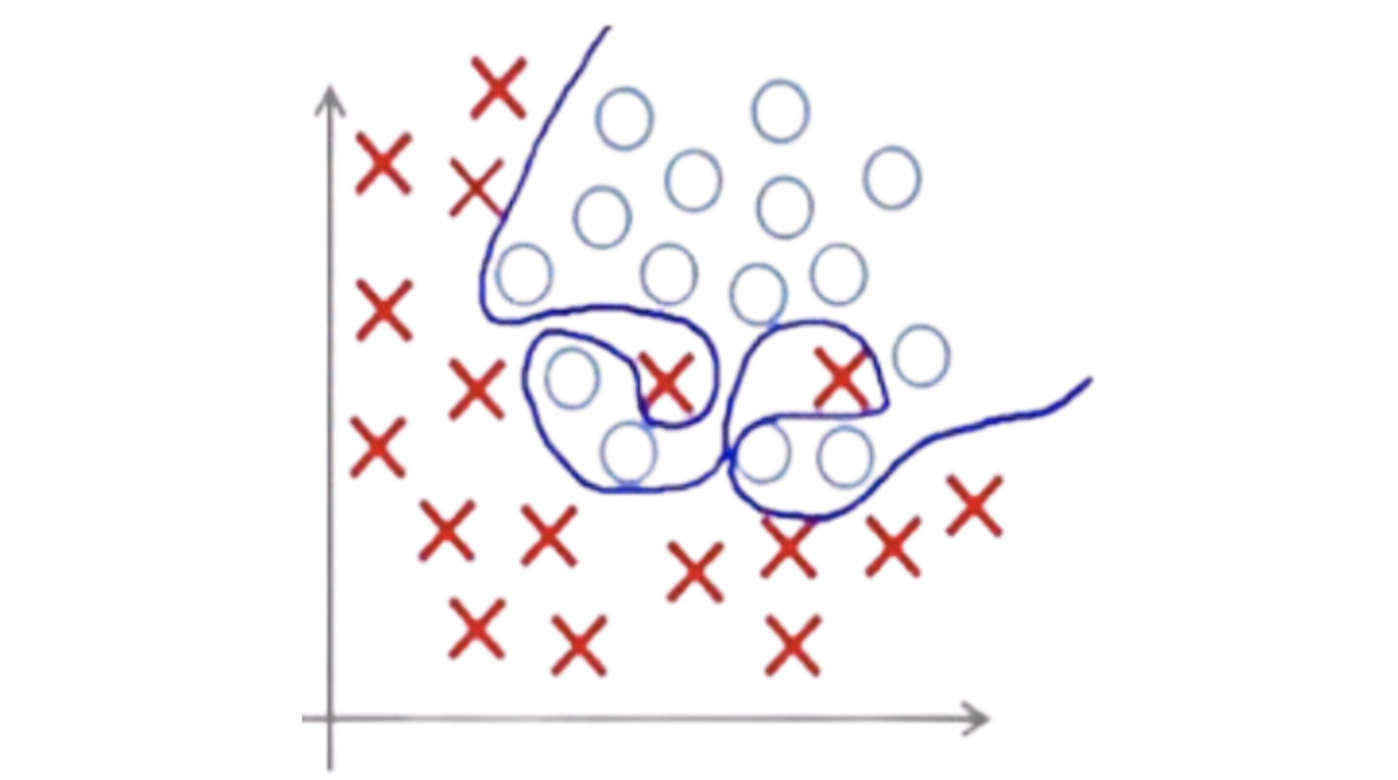#### Possible reasons and cures for overfitting

The possible reasons could be,

• ML model is learning everything present in the data, even the noises.
• The model that we have chosen is unnecessarily stronger.

Here, increasing data can help bring diversity in the data samples, and the model will learn the generalizability. But getting more data sometimes can be infeasible. In such scenarios, we can try to reduce the model complexity and check the performance or use the regularization techniques, which we will discuss in this blog.

### Appropriate Fitting

This type of fitting is considered the best fitting where training error is slightly higher for training data when compared to overfitting, but testing error reduces significantly for testing data.

#### How to detect appropriate fitting?

In this case, the gap between human error and training error becomes equal or higher than the gap present at the time of overfitting. But the gap between training error and the test error becomes significantly smaller.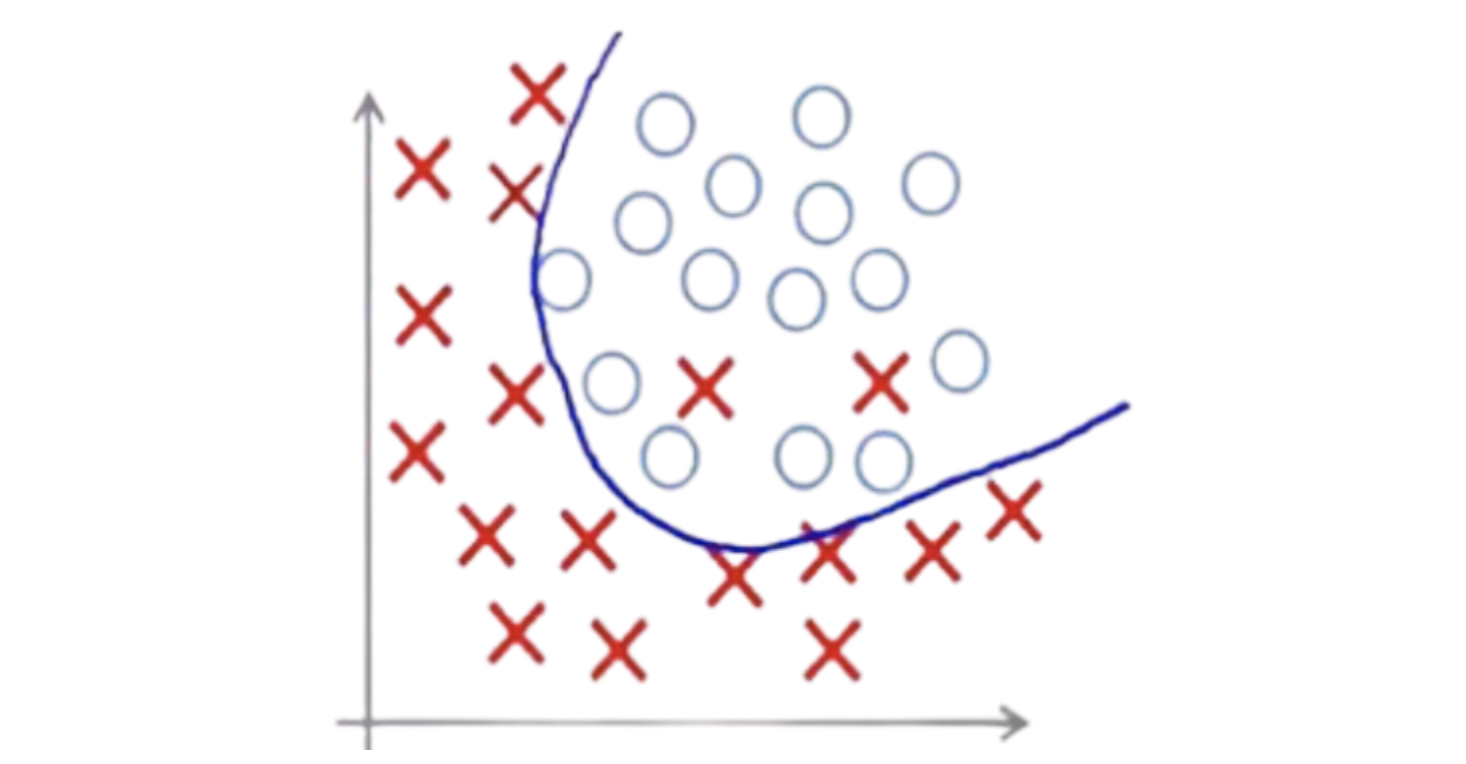Let’s take an example to learn it better. Suppose we have been provided the data for the Price of the vehicle vs. the size of the vehicle. In the figure below, green X represents the given data in a 2D plane. We can say that any quadratic function can appropriately fit the given data, but machine learning algorithms are unaware. They can not say it before actually fitting it.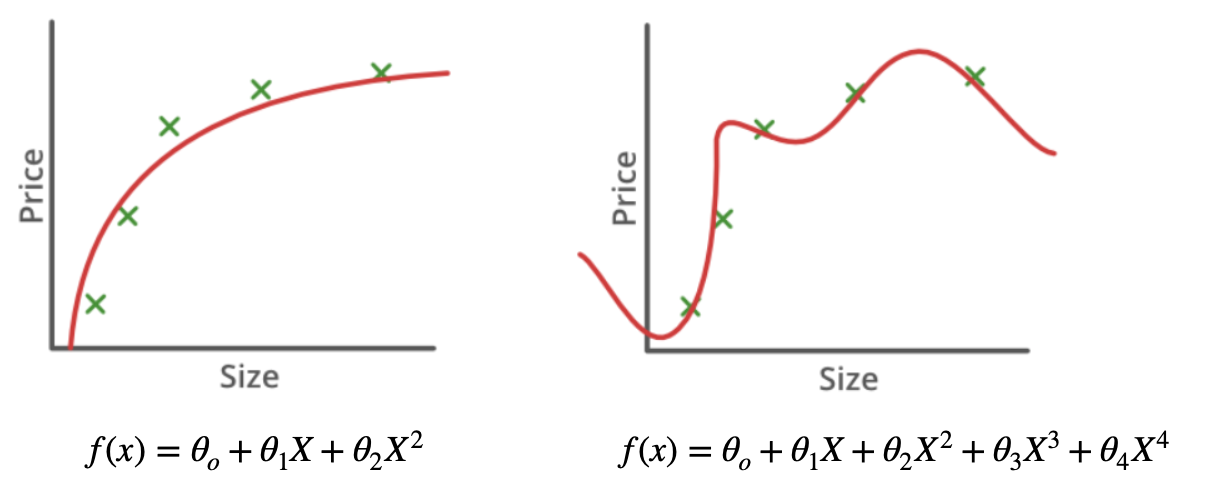Figure 1

We know that the overall objective of a machine learning algorithm is to reduce the error between predicted and actual values. Mathematically we can represent our objective as minimize the sum of squares of all the prediction errors. We can say that this is our cost function.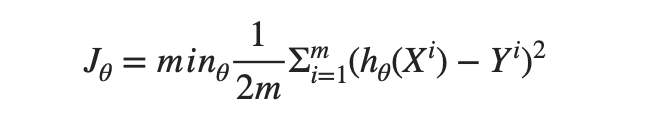hθ(Xi) gives prediction by the machine learning model for an ith sample of input, and we have m such samples.

If our model fits a higher degree polynomial (Let’s say 4th order) to the given data, the error will be minimum, and we can say the model will overfit the data. We already know that overfitting is not good for a machine learning model, and hence we need to figure out a way such that fitting happens up to quadratic order only.

What if we make values of θ2 and θ3 (See figure 1) extremely small so that X³ and X⁴ do not contribute significantly to the final fitting.

Can you think how can we do that ?

Yes! Absolutely there is a way. Let’s modify our cost function below, where 1000 is just any random huge number. Modified cost function will look like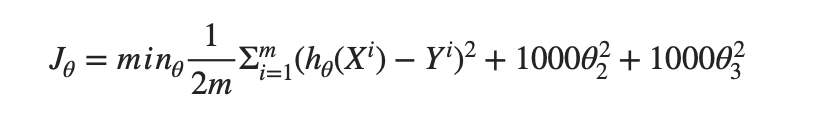As we know, our machine learning algorithms are trying to reduce the cost function. To achieve the minimum of the modified cost function, θ2 and θ3 will be given minimal values.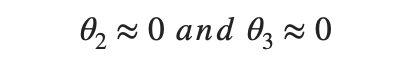Which eventually will convert our 4th order polynomial to 2nd order polynomial, and the problem of overfitting will be resolved.From the previous example, if we observe, two main objectives got fulfilled after penalizing the irrelevant parameters,

1. The order of the curve that is to be fitted got simpler.
2. Overfitting reduced

Regularization is the concept that is used to fulfill these two objectives mainly.

Suppose there are a total of n features present in the data. Our Machine Learning model will correspondingly learn n + 1 parameters, i.e.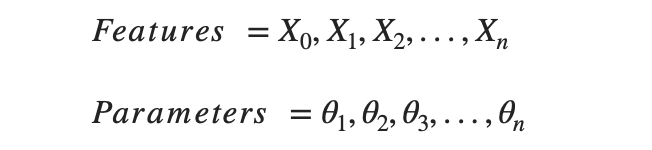We can easily penalize the corresponding parameters if we know the set of irrelevant features, and eventually, overfitting will be reduced. But finding that set of irrelevant features is not possible.

To overcome this problem, penalization is applied to every parameter. After penalization, our new cost function would be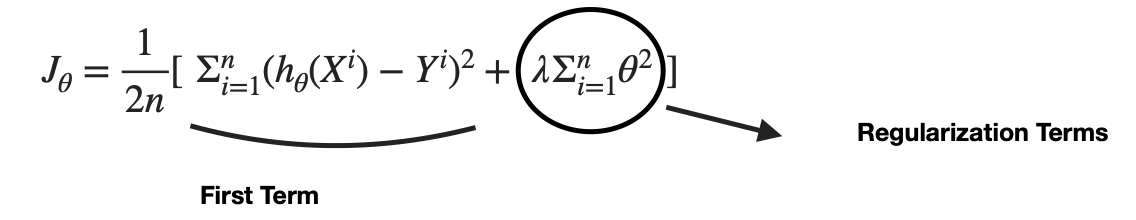Figure 2

The new cost function controls the tradeoff between two goals,

1. Fitting the training set well, which is ensured by the First term from the above formulae
2. Keeping the parameters small so that the hypothesis becomes simpler, which is ensured by the regularization term. λ is the regularization parameter.

One question that must come to our mind now, that

What if we set a huge value of λ in our cost function, let’s say 10¹⁰?

To understand this, let’s take the same above curve fitting example where our model fitted a 4th order polynomial,If we make the λ value very high, then every parameter will become very small, I.e.And ML would fit a linear line, f(x) = θo, a perfect case of underfitting. Hence, we need to choose the value of lambda wisely.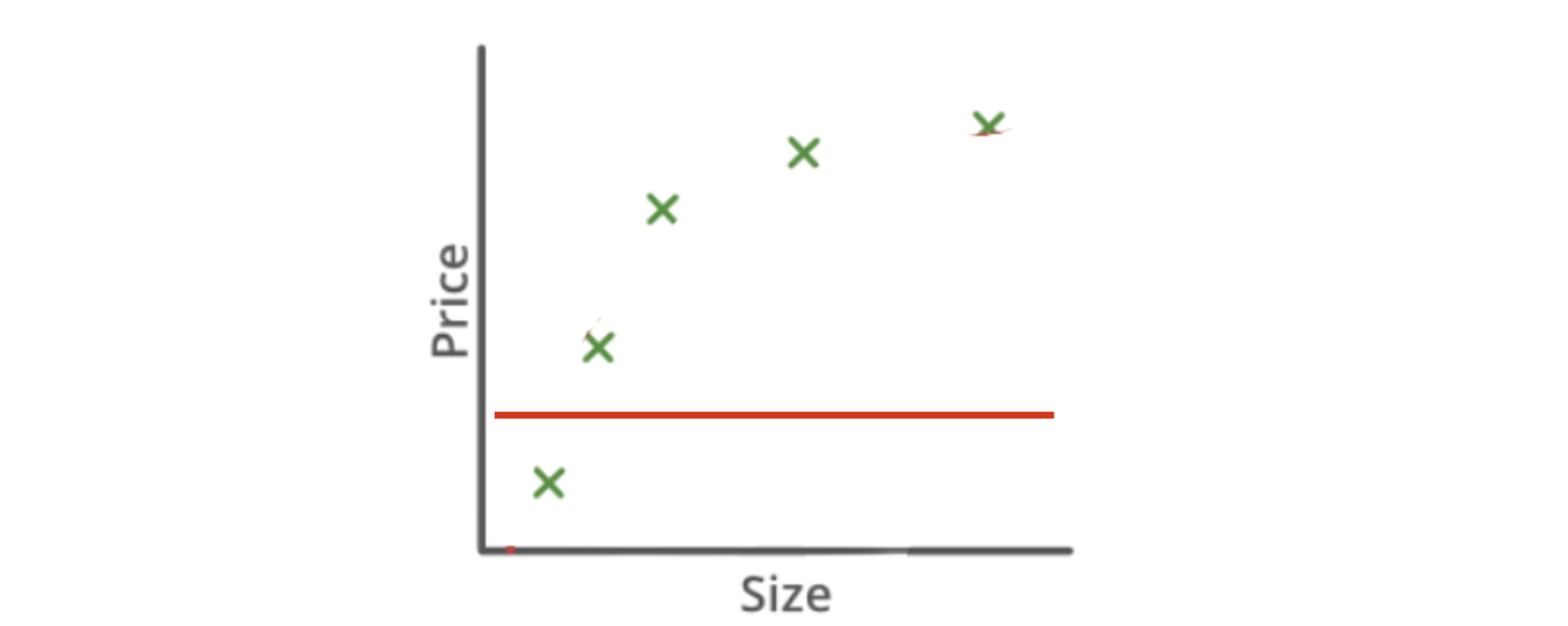To achieve the minima of the cost function faster, we use optimizers that consistently navigate the parameter updation steps. We consecutively update the values of different parameters and check the cost function minima.

Let’s update the parameter using the Gradient Descent optimizer method and see how this regularization parameter is working,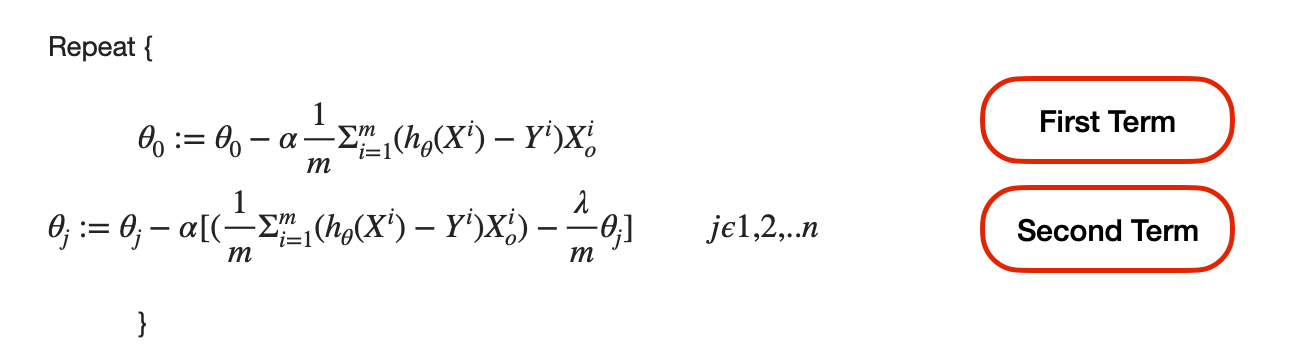To observe closely, rearrange the second term,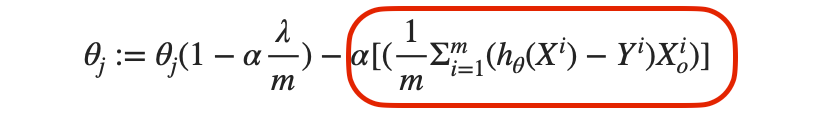As α (learning rate), λ, and m are all positive, hence in every iteration, θj is reducing. At each iteration, we are checking whether the cost function is decaying or not. As regularisation parameter affects the cost function and updation of parameters, it penalizes those parameters that derive the model in the wrong direction. Eventually, the reduction of those θjs happens more, and automatically the dimensions corresponding to that θ become less significant. Via this way, regularization solves the problem of overfitting.

Now, as we know the question of “how”, let’s move ahead and learn the two most common regularization methods used in linear/logistic regression,

#### L1-Regularization

Adds “absolute value of magnitude” of coefficient as penalty term to the loss function.This is also known as the LASSO (Least Absolute Shrinkage and Selection Operator) regression. Lasso shrinks the coefficient of irrelevant or less important features to zero and eventually helps in feature selection.

#### L-2 Regularization

Adds “squared magnitude” of coefficient as penalty term to the loss function.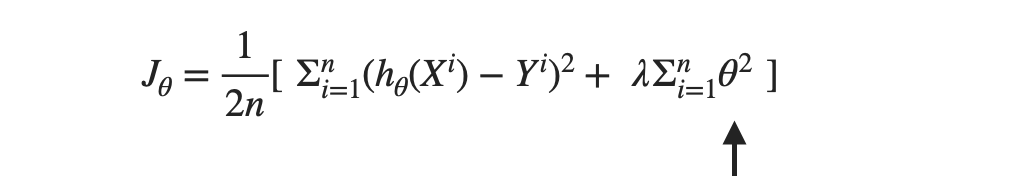Regression models that use L2-regularization are also known as Ridge regression.

#### Possible interview questions on this topic

This is one of the hottest topics in machine learning on which you will definitely be questioned in machine learning interviews. Some possible ones are:

1. What is underfitting or overfitting?
2. How will you decide that your model is underfitting or overfitting?
3. How will you cure the problem of underfitting?
4. How will you cure the problem of overfitting?
5. What are the regularization techniques used in ML?
6. What’s the difference between L1 and L2 regularization techniques?

#### Conclusion

Overfitting is one of the most easily found problems while addressing any problem statement using machine learning models. Regularization techniques are the cure for overfitting. In this article, we have discussed how regularization techniques cure the problem of overfitting. We also discussed the possible reasons for underfitting and overfitting and what can be done to eliminate these problems. This topic is one of the hottest topics in machine learning interviews, and we hope we made it clear to you.

### Enjoy Learning! Enjoy Algorithms!

Get well-designed application and interview centirc content on ds-algorithms, machine learning, system design and oops. Content will be delivered weekly.